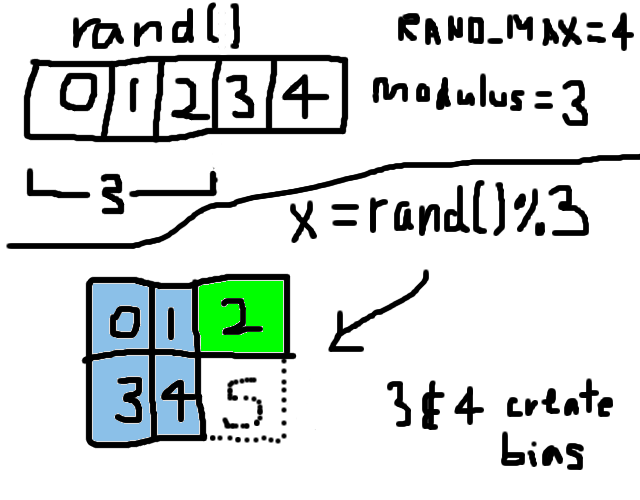# Generating Random Numbers without Modulo Bias

UPDATE: See this blog post.

Let’s say you need a random number from 0 to 2 (0, 1, or 2). If you do this:

/* Listing 1 */
int n, x;
n = 3;
x = rand() % n;
return x;

your code is broken, thanks to modulo bias. You will always get modulo bias (however slight, or great, it may be) whenever RAND_MAX + 1 of your rand() function is not evenly divisible by your modulus n.

Continuing with our example, let’s say rand()’s RAND_MAX is 4, so there are 5 different possible values that rand() can generate (0, 1, 2, 3, or 4). Now rand() will return each value 20% of the time, assuming that rand() has very good distribution from 0 to RAND_MAX.1 But since we take the modulo by 3, we get 0 40% of the time (0 and 3)! This fact alone should make you worried. Visually it is as follows:The following code is correct:

/* Listing 2 */
int n, x, rand_limit, rand_excess;
n = 3;
rand_excess = (RAND_MAX + 1) % n;
rand_limit = RAND_MAX - rand_excess;
while (x = rand() > rand_limit) {};
return x % n;

So, if RAND_MAX is 4 as in the previous example, rand_excess is $(4 + 1) \mbox{ mod 3}\\ = 5 \mbox{ mod 3}\\ = 2$. Then rand_limit becomes $4 - 2 = 2$. The while statement then throws out the values 3 and 4 (i.e., x is only allowed to be 0, 1, or 2). Then, we return the modulo expression x % n (which is redundant here onlyu because RAND_MAX is very low for the sake of our example).

A problem might exist if RAND_MAX is already the maximum value allowed by the type that rand() returns. E.g., if rand() returns a 64-bit unsigned integer (in C99, this is the uint64_t type) , and RAND_MAX is already set to $2^{64} - 1$, then RAND_MAX - 1 would wrap back around to 0, and rand_excess would likewise be 0. To avoid this, you can use the alternate expression rand_excess = (RAND_MAX % n) + 1; for setting rand_excess:

/* Listing 3 */
int n, x, rand_limit, rand_excess;
n = 3;
rand_excess = (RAND_MAX % n) + 1; /* only difference vs listing-2.c */
rand_limit = RAND_MAX - rand_excess;
while (x = rand() > rand_limit) {};
return x % n;

This way, you ensure that you shrink the value of RAND_MAX to something smaller first, before adding 1 to it as in Listing 2. Unfortunately, there still remains a problem with this workaround: there is a remote chance that rand_excess will be equal to n, needlessly reducing the size of rand_limit (and thus throwing away perfectly bias-free numbers generated by rand()). For example, say RAND_MAX is 8 and n is 3. Then rand_excess is $(8 \mbox{ mod 3}) + 1\\ = 2 + 1\\ = 3$. Now rand_limit is $8 - 3 = 5$. But RAND_MAX of 8 is already valid because there are 9 possible values 0 through 8, and there is no modulo bias to begin with (since $9 \mbox{ mod 3} = 0$)! To get around this, we can do one more modulo operation rand_excess = ((RAND_MAX % n) + 1) % n; to avoid the case where rand_excess could equal n:

/* Listing 4 */
int n, x, rand_limit, rand_excess;
n = 3;
rand_excess = ((RAND_MAX % n) + 1) % n; /* only difference vs listing-3.c */
rand_limit = RAND_MAX - rand_excess;
while (x = rand() > rand_limit) {};
return x % n;

So if RAND_MAX is 8 and n is 3, then rand_excess is $((8 \mbox{ mod 3}) + 1) \mbox{ mod 3}\\ = (2 + 1) \mbox{ mod 3}\\ = 3 \mbox{ mod 3}\\ = 0$ and rand_limit would not be reduced by any amount.2

If you actually know beforehand the exact size of RAND_MAX (usually it is INT_MAX or some such, determined by the type of the variable returned by rand()), you should instead just define rand_limit as a constant to save some CPU cycles (write out the mathematical expressions involved by hand to find the number and just hardcode it).

1. All modern rand() functions should have very good distribution.↩︎

2. Thanks to Stanislav Tsyganov for this improvement over Listing 3.↩︎